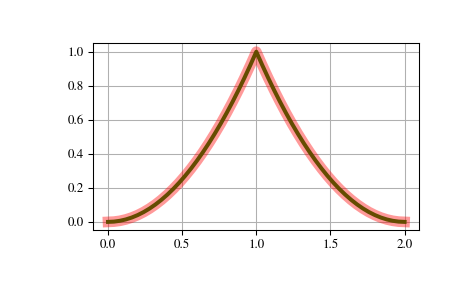# scipy.interpolate.BSpline.basis_element¶

classmethod BSpline.basis_element(t, extrapolate=True)[source]

Return a B-spline basis element B(x | t, ..., t[k+1]).

Parameters: t : ndarray, shape (k+1,) internal knots extrapolate : bool or ‘periodic’, optional whether to extrapolate beyond the base interval, t .. t[k+1], or to return nans. If ‘periodic’, periodic extrapolation is used. Default is True. basis_element : callable A callable representing a B-spline basis element for the knot vector t.

Notes

The order of the b-spline, k, is inferred from the length of t as len(t)-2. The knot vector is constructed by appending and prepending k+1 elements to internal knots t.

Examples

Construct a cubic b-spline:

>>> from scipy.interpolate import BSpline
>>> b = BSpline.basis_element([0, 1, 2, 3, 4])
>>> k = b.k
>>> b.t[k:-k]
array([ 0.,  1.,  2.,  3.,  4.])
>>> k
3


Construct a second order b-spline on [0, 1, 1, 2], and compare to its explicit form:

>>> t = [-1, 0, 1, 1, 2]
>>> b = BSpline.basis_element(t[1:])
>>> def f(x):
...     return np.where(x < 1, x*x, (2. - x)**2)

>>> import matplotlib.pyplot as plt
>>> fig, ax = plt.subplots()
>>> x = np.linspace(0, 2, 51)
>>> ax.plot(x, b(x), 'g', lw=3)
>>> ax.plot(x, f(x), 'r', lw=8, alpha=0.4)
>>> ax.grid(True)
>>> plt.show()#### Previous topic

scipy.interpolate.BSpline.__call__

#### Next topic

scipy.interpolate.BSpline.derivative